# GMAT Math : Specific Value Data Sufficiency

## Example Questions

### Example Question #1321 : Data Sufficiency Questions

A beer company spent $100,000 last year on hops, yeast, and malt. How much of the total expenditure was for hops? 1. The expenditure for yeast was 20% greater than the expenditure for malt. 2. The total expenditure for yeast and malt was equal to the expenditure for hops. Possible Answers: Both statements (1) and (2) TOGETHER are sufficient to answer the question asked; but NEITHER statement ALONE is sufficient EACH statement ALONE is sufficient to answer the question asked Statements (1) and (2) TOGETHER are NOT sufficient to answer the question asked, and additional data specific to the problem are needed Statement (2) ALONE is sufficient, but statement (1) alone is not sufficient to answer the question asked Statement (1) ALONE is sufficient, but statement (2) alone is not sufficient to answer the question asked Correct answer: Statement (2) ALONE is sufficient, but statement (1) alone is not sufficient to answer the question asked Explanation: A crucial strategy for Data Sufficiency problems is making sure to leverage all of the information given in the question stem. Here the fact that hops, yeast, and malt expenditures total$100,000 allows you to begin - before you ever get to the statements - with the equation:

H + Y + M = $100,000 When you then combine this with statement 1, you should see that the information remains insufficient. Statement 1 can be expressed as Y = 1.2M, allowing you to streamline the given equation to: H + 1.2M + M =$100,000

But since you still have two variables you cannot solve for either. Since statement 1 is insufficient you can eliminate choices "Statement (1) ALONE is sufficient, but statement (2) alone is not sufficient to answer the question asked" and "EACH statement ALONE is sufficient to answer the question asked".

When you combine the given information with statement 2, however, note that you have:

Given: H + Y + M = $100,000 Statement 2: Y + M = H This then lets you substitute for the expression Y + M in the initial equation, giving you: H + H =$100,000

Since 2H = $100,000, that means that H =$50,000. Since the question asks you for H, you have sufficient information. Choice "Statement (2) ALONE is sufficient, but statement (1) alone is not sufficient to answer the question asked" is correct.

### Example Question #1324 : Data Sufficiency Questions

Jeff’s average (arithmetic mean) monthly utility bill for the months of June, July, and August was $75. During which month did he receive the highest bill? 1. The lowest bill was received in July. 2. The total of his bills for July and August was$160.

Both statements (1) and (2) TOGETHER are sufficient to answer the question asked; but NEITHER statement ALONE is sufficient

Statements (1) and (2) TOGETHER are NOT sufficient to answer the question asked, and additional data specific to the problem are needed

Statement (1) ALONE is sufficient, but statement (2) alone is not sufficient to answer the question asked

Statement (2) ALONE is sufficient, but statement (1) alone is not sufficient to answer the question asked

Both statements (1) and (2) TOGETHER are sufficient to answer the question asked; but NEITHER statement ALONE is sufficient

Explanation:

As you assess this question, first note the given information in the question stem: the average for the 3 months was $75. Since the question requires you to separate the three months' bills to determine which is highest, it is a good idea to just multiply this average by the number of terms (3) right away to get the total amount for the 3 months:$225 total.

Statement 1 tells you that the lowest bill was received in July. This means that the July bill must be lower than $75, however, the highest bill could still be for June or August. Since this is not specific it is not sufficient. Eliminate choices "Statement (1) ALONE is sufficient, but statement (2) alone is not sufficient to answer the question asked" and "EACH statement ALONE is sufficient to answer the question asked". Statement 2 tells you that the total for July and August was$160. This gives you the exact amount for June. $225 – 160 =$65 for June. But remember - at this point you are only assessing statement 2 alone, so be careful not to include the information from statement 1 at this point. You do not know if July or August is the highest bill. This statement alone is not sufficient. Eliminate choice "Statement (2) ALONE is sufficient, but statement (1) alone is not sufficient to answer the question asked".

When you take the statements together you know that the June bill is $65 and that the July bill is less than this amount. Clearly August is the highest bill since both June and July are below the average. Because the statements together (but not alone) are sufficient, the correct answer is "Both statements (1) and (2) TOGETHER are sufficient to answer the question asked; but NEITHER statement ALONE is sufficient". ### Example Question #1325 : Data Sufficiency Questions A certain company paid bonuses of$125 to each of its executive employees and $75 to each of its non-executive employees. If 100 of the employees were non-executives, how many of the employees were executives? 1. The company has a total of 120 employees. 2. The total amount that the company paid in bonuses to its employees was$10,000.

Statements (1) and (2) TOGETHER are NOT sufficient to answer the question asked, and additional data specific to the problem are needed

Statement (2) ALONE is sufficient, but statement (1) alone is not sufficient to answer the question asked

Both statements (1) and (2) TOGETHER are sufficient to answer the question asked; but NEITHER statement ALONE is sufficient

Statement (1) ALONE is sufficient, but statement (2) alone is not sufficient to answer the question asked

Explanation:

For all “what is the value” data sufficiency questions, you are looking for consistency in the value you are asked to find. Remember - that means that you don’t necessarily have to solve for the value in question, so often you can leave these questions one step short if you recognize that an equation has only one solution.

In this question, you are asked for the total number of executives, given that each employee was given a bonus ($75 for non-executives and$125 for executives) and that there were 100 non-executives. Notice that the wording of the problem means that there can only be two categories – people who are executives and people who are not. That means that there aren’t other groups that aren’t accounted for.

Statement (1) gives that there are 120 total employees. Since you know that there are 100 non-executives, that means that you can set up the simple equation

100 + E = 120, where E is the number of executives.

Because this is a one-variable equation, statement (1) is obviously sufficient. Eliminate "Statement (2) ALONE is sufficient, but statement (1) alone is not sufficient to answer the question asked", "Both statements (1) and (2) TOGETHER are sufficient to answer the question asked; but NEITHER statement ALONE is sufficient", and "Statements (1) and (2) TOGETHER are NOT sufficient to answer the question asked, and additional data specific to the problem are needed".

Statement (2) gives that the company gave a total of $10,000 in bonuses, you can set up and solve another single-variable equation, since you know that Total Bonus Amount = (100)(75) + (E)(125) Since you know that there are 100 non-executives and that they gave a total of$10,000 in bonuses, you can plug in to the equation to get:

10,000 = (100)(75) + (E)(125)

Since you have a single-variable linear equation, you know that if you solve you will get only one value for E. You already know, then, that statement (2) is sufficient and can leave the equation unsolved. Therefore, eliminate "Statement (1) ALONE is sufficient, but statement (2) alone is not sufficient to answer the question asked" and choose "EACH statement ALONE is sufficient to answer the question asked" – both statements are independently sufficient.

### Example Question #1326 : Data Sufficiency Questions

A certain jewelry store sells gold necklaces in 18-inch and 28-inch lengths, and all necklaces of the same length sell for the same price per necklace regardless of the number of necklaces purchased. What is the price of a 28-inch necklace at this jewelry store?

1. The total price of an 18-inch and a 28-inch gold necklace is $68. 2. The total price of two 18-inch necklaces and one 28-inch necklace is$96.

Statement (1) ALONE is sufficient, but statement (2) alone is not sufficient to answer the question asked

Statements (1) and (2) TOGETHER are NOT sufficient to answer the question asked, and additional data specific to the problem are needed

Both statements (1) and (2) TOGETHER are sufficient to answer the question asked; but NEITHER statement ALONE is sufficient

Statement (2) ALONE is sufficient, but statement (1) alone is not sufficient to answer the question asked

Both statements (1) and (2) TOGETHER are sufficient to answer the question asked; but NEITHER statement ALONE is sufficient

Explanation:

Any time you are set up questions for a problem on the GMAT, remember that you should pick meaningful variables that are clearly tied to the problem you are working with. In this case, instead of “x” and “y”, you can pick “S” for the shorter (18-inch) chain and “L” for the longer (28-inch) chain.

Your question can then be written as “What is the value of L?” You know from the information given in the prompt that S and L have only one value each since there is no such thing as a “volume” discount for buying in bulk, so if you can solve for a single, consistent answer for L, you know that a statement must be sufficient.

Statement (1) gives that the total price for buying one 18-inch and one 28-inch chain is $68. If you translate this into an equation, this gives you S + L = 68. Since this is a two-variable equation, it is impossible to solve for L without additional information since there are multiple possible combinations for values of S and L. For example, S = 12 and L = 56 would satisfy the equation, but so would S = 1 and L = 67. Statement (1) is therefore insufficient – eliminate "Statement (1) ALONE is sufficient, but statement (2) alone is not sufficient to answer the question asked" and "EACH statement ALONE is sufficient to answer the question asked". Statement (2) gives that the price of two 18-inch necklaces and one 28-inch necklace is$98. If you translate this into an equation, this gives you

2S + L = 98

Again, since you are left with a two-variable equation and no additional information, it is impossible to solve for S or L. Eliminate "Statement (2) ALONE is sufficient, but statement (1) alone is not sufficient to answer the question asked".

Taken together, the two statements give you a system of linear equations:

S + L = 68

2S + L = 98

You may be tempted to go ahead and solve this, but remember that sufficiency requires consistency, not an answer. So ask yourself: what condition needs to be met in order for a system of equations to have only one answer? The two variables must be in different ratios in the two different equations. So in this case, S and L are in a 1:1 ratio in the first equation and a 2:1 ratio in the second. Because these are different, you can conclude that system will yield an answer and that the statements are sufficient together and pick (C).

If this wasn’t immediately obvious, you could always solve the system of equations by negating the first equation and adding:

-S – L = - 68

+2S + L = 98

Which yields S = 30. Substituting back into the first equation then gives you that L = 38.

The correct answer is "Both statements (1) and (2) TOGETHER are sufficient to answer the question asked; but NEITHER statement ALONE is sufficient".

### Example Question #1327 : Data Sufficiency Questions

For integersand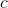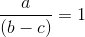.What is the value of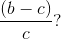1.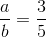2.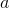and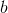have no common factors greater than 1.

Both statements (1) and (2) TOGETHER are sufficient to answer the question asked; but NEITHER statement ALONE is sufficient

Statements (1) and (2) TOGETHER are NOT sufficient to answer the question asked, and additional data specific to the problem are needed

Statement (1) ALONE is sufficient, but statement (2) alone is not sufficient to answer the question asked

Statement (2) ALONE is sufficient, but statement (1) alone is not sufficient to answer the question asked

Statement (1) ALONE is sufficient, but statement (2) alone is not sufficient to answer the question asked

Explanation:

For data sufficiency questions that give multiple algebraic expressions, it is usually easier and more accurate to perform some algebraic manipulation to “make your math look like theirs” than it is to pick numbers. Remember, algebra doesn’t lie, and leveraging your assets can make the question stem easier to evaluate.

The question stem gives thatand asks for the value of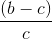Notice the similarities between the two fractions – it is possible to leverage this information to simplify the question stem.

If you take the first equation and multiply both sides by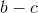, you get: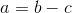You can then substitutein forin the question stem to get

What is the value of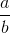? – a much simpler question to deal with.

Statement (1) is then automatically recognizable as sufficient – it gives a value for. There is no room for any other value for the fraction, so this is sufficient. Eliminate "Statement (2) ALONE is sufficient, but statement (1) alone is not sufficient to answer the question asked", "Both statements (1) and (2) TOGETHER are sufficient to answer the question asked; but NEITHER statement ALONE is sufficient", and "Statements (1) and (2) TOGETHER are NOT sufficient to answer the question asked, and additional data specific to the problem are needed".

Statement (2) gives thatandhave no common factors greater than 1. This means that bothandare either primes or have no prime factors in common. However, it gives no other information. You can quickly prove this insufficient by picking numbers:

It is possible thatandare 3 and 5 respectively and thatHowever, it is also possible thatandare 7 and 9 and that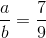Statement (2) is therefore insufficient, eliminating "EACH statement ALONE is sufficient to answer the question asked" and leaving you with answer choice "Statement (1) ALONE is sufficient, but statement (2) alone is not sufficient to answer the question asked".

### Example Question #1328 : Data Sufficiency Questions

By how many years is Jason older than Allison?

(1) Jason is 6 years older than twice David’s age.

(2) In 8 years, Allison will be twice as old as David is now.

Both statements (1) and (2) TOGETHER are sufficient to answer the question asked; but NEITHER statement ALONE is sufficient

Statement (2) ALONE is sufficient, but statement (1) alone is not sufficient to answer the question asked

Statements (1) and (2) TOGETHER are NOT sufficient to answer the question asked, and additional data specific to the problem are needed

Statement (1) ALONE is sufficient, but statement (2) alone is not sufficient to answer the question asked

Both statements (1) and (2) TOGETHER are sufficient to answer the question asked; but NEITHER statement ALONE is sufficient

Explanation:

An important Data Sufficiency strategy is to always note when a question is asking you about a combination of variables (here that's "What is J - A?") as opposed to a single variable (either J or A). Almost always, as is the case here, it requires less information to solve directly for the combination than it does to solve for the individual variables and then combine yourself.

Note that neither statement allows you to assess both J and A, so the answer cannot be "Statement (1) ALONE is sufficient, but statement (2) alone is not sufficient to answer the question asked", "EACH statement ALONE is sufficient to answer the question asked", or "Statement (2) ALONE is sufficient, but statement (1) alone is not sufficient to answer the question asked". But when you take the two statements together, you have:

(1) J = 6 + 2D

(2) A + 8 = 2D

If you then subtract the entire second equation from the first, you'll combine:

J = 6 + 2D -A - 8 = -2D

To get:

J - A - 8 = 6

So when you add 8 to both sides you're finished:

J - A = 14

Because you can solve directly for J - A, even though you do not know the individual variables J or A, this is sufficient information and the correct answer is "Both statements (1) and (2) TOGETHER are sufficient to answer the question asked; but NEITHER statement ALONE is sufficient".

### Example Question #1321 : Data Sufficiency Questions

Exactly how many show dogs does Sheila have?

1. Of Sheila’s show dogs, exactly 3 have won prizes of at least $500. 2. Of Sheila’s show dogs, exactly 40% have not won a prize of$500 or more.

Statement (2) ALONE is sufficient, but statement (1) alone is not sufficient to answer the question asked

Statements (1) and (2) TOGETHER are NOT sufficient to answer the question asked, and additional data specific to the problem are needed

Both statements (1) and (2) TOGETHER are sufficient to answer the question asked; but NEITHER statement ALONE is sufficient

Statement (1) ALONE is sufficient, but statement (2) alone is not sufficient to answer the question asked

Both statements (1) and (2) TOGETHER are sufficient to answer the question asked; but NEITHER statement ALONE is sufficient

Explanation:

This question asks for the specific number of show dogs. As with any "what is the value" data sufficiency question, a sufficient statement will ensure that there is one and only one possible value for the number of show dogs. Remember if you are picking numbers to test a statement that just because a statement gives an answer doesn't mean that it gives only ONE answer.

Statement (1) states that “Exactly 3 of the dogs won prizes of at least $500.” This statement is not sufficient alone. Consider a few scenarios: the three dogs that won show prizes could be her only dogs, or Sheila could have 100 dogs - 97 that have not one prizes of at least$500 and 3 that have. There is no way for you to determine the total number of show dogs. Eliminate choices "Statement (1) ALONE is sufficient, but statement (2) alone is not sufficient to answer the question asked" and "EACH statement ALONE is sufficient to answer the question asked".

Statement (2) states that “40% of the dogs have not won a prize of $500 or more.” Using this information, you should recognize that this means that 60% of her dogs have won a prize of$500 or more. However, this statement is also not sufficient alone since there is no information about what value you are taking a percentage of.

In evaluating (2), remember that you can't bring down any information from statement (1), so Sheila may not have just 3 dogs that have won prizes. Sheila could have 100 dogs, 60 of which have won prizes, or 10 dogs, six of which have won prizes. As with statement (1), there is no way to determine the total number of dogs. Eliminate choice "Statement (2) ALONE is sufficient, but statement (1) alone is not sufficient to answer the question asked".

Taking the two statements, together, you know from statement (1) that 3 dogs have won prizes of $500 or more and you know from statement (2) that 60% of the dogs have won a prize of$500 or more.

You can use these two statements to set up and solve a single-variable equation, since those two statements must equal the same value. So that means “3 dogs = 60% of the total dogs” or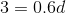You can either recognize that this is sufficient since it will yield only one value for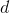, or go ahead and solve this equation by dividing both sides by 0.6. If you solve for, you'll find that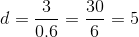, so she has 5 show dogs total. This information is sufficient and the correct answer is therefore "Both statements (1) and (2) TOGETHER are sufficient to answer the question asked; but NEITHER statement ALONE is sufficient".

### Example Question #1321 : Data Sufficiency Questions

Julie runs a small art museum. How many paintings does her museum have?

1. Her next acquisition of 4 Monet paintings will increase the number of paintings in the museum’s collection by 10%.
2. The museum currently exhibits 3 impressionist paintings for every 2 non-impressionist paintings.

Statement (1) ALONE is sufficient, but statement (2) alone is not sufficient to answer the question asked

Statement (2) ALONE is sufficient, but statement (1) alone is not sufficient to answer the question asked

Statements (1) and (2) TOGETHER are NOT sufficient to answer the question asked, and additional data specific to the problem are needed

Both statements (1) and (2) TOGETHER are sufficient to answer the question asked; but NEITHER statement ALONE is sufficient

Statement (1) ALONE is sufficient, but statement (2) alone is not sufficient to answer the question asked

Explanation:

This question asks you to provide a specific number of paintings in the museum. Since this is a "what is the value" data sufficiency question, remember that you are looking for each statement to give you a consistent value for the number of paintings. If any statement gives multiple possible values, it is insufficient. Additionally, remember to always pick descriptive variables that can clearly be associated with what you're solving for.

Statement (1) states that adding 4 new paintings to the collection will increase the number of paintings in the museum by 10%. You should then recognize that if adding 4 paintings increases the size of the collection by 10%, you can plug in the values you know into what you know about percent change:

New value = (1 + percent change)(Original value)

In the context of this problem, this can be rewritten as

P + 4 = 1.1P,

where P equals the total number of paintings. Because you now have a single variable equation with only one solution, this information will be sufficient to provide a specific answer to the question. There is no need to actually solve this equation. Statement (1) is sufficient, meaning that you can eliminate "Statement (2) ALONE is sufficient, but statement (1) alone is not sufficient to answer the question asked", "Both statements (1) and (2) TOGETHER are sufficient to answer the question asked; but NEITHER statement ALONE is sufficient", and "Statements (1) and (2) TOGETHER are NOT sufficient to answer the question asked, and additional data specific to the problem are needed".

Statement (2) gives that the ratio of impressionist to non-impressionist paintings is 3:2. This information does not allow you to arrive at a specific number since you do not know either the number of impressionist or the number of non-impressionist paintings. There could be 3 impressionist and 2 non-impressionist paintings for a total of 5 paintings. However, because you only have a ratio, the total could also be any multiple of 5 as long as the numbers of non-impressionist and impressionist paintings are in the correct ratio.

Because statement (2) returned two unique values for the number of paintings, it is not sufficient. The answer is "Statement (1) ALONE is sufficient, but statement (2) alone is not sufficient to answer the question asked".

### Example Question #2 : Specific Value Data Sufficiency

A bake sale sells only individual cupcakes and boxes of cookies. If cupcakes sell for $2 each, and a box of cookies sells for$15, how many boxes of cookies did Kevin buy?

(1) Kevin spent $27 (2) Kevin bought 6 cupcakes Possible Answers: EACH statement ALONE is sufficient to answer the question asked Both statements (1) and (2) TOGETHER are sufficient to answer the question asked; but NEITHER statement ALONE is sufficient Statement (1) ALONE is sufficient, but statement (2) alone is not sufficient to answer the question asked Statement (2) ALONE is sufficient, but statement (1) alone is not sufficient to answer the question asked Statements (1) and (2) TOGETHER are NOT sufficient to answer the question asked, and additional data specific to the problem are needed Correct answer: Statement (1) ALONE is sufficient, but statement (2) alone is not sufficient to answer the question asked Explanation: Because this is a "what is the value" data sufficiency problem, for a statement to be sufficient it must yield one and only one value for the number of boxes of cookies Kevin bought. Any time you have a data sufficiency problem couched in real-world terms, think about what the implications of the set up are. In this case, Keven can only buy whole numbers of cupcakes and boxes of cookies. This is important since it limits the number of combinations within the problem. According to the question stem, you know that Kevin bought an unknown number of cupcakes and boxes of cookies for$2 and $15 each, respectively. Statement 1 tells you that Kevin spent$27 total. Cupcakes sell for an even dollar amount, and a box of cookies sells for an odd dollar amount. Kevin cannot have purchased ONLY cupcakes and still have an odd total, since an even number plus an even number will always be even. That means that he must have bought at least one box of cookies.

If he bought one box of cookies for $15, he would have had$12 left over to buy 6 total cupcakes.

However, could he have bought more than one box of cookies? Assume that Kevin bought two boxes of cookies for $15 each and spent$30. There are two problems. First, the number is even, not odd. However, second (and most importantly) the question stem states that Kevin only spent $27, so he can't have spent$30 on two boxes of cookies!

Because there is therefore only one valid combination of cookies and cupcakes, we can determine that he purchased exactly ONE box of cookies. Statement 1 is therefore sufficient. Eliminate "Statement (2) ALONE is sufficient, but statement (1) alone is not sufficient to answer the question asked", "Both statements (1) and (2) TOGETHER are sufficient to answer the question asked; but NEITHER statement ALONE is sufficient", and "Statements (1) and (2) TOGETHER are NOT sufficient to answer the question asked, and additional data specific to the problem are needed".

Statement 2 gives that Kevin purchased 6 cupcakes. However, without any information about how much money spent in total, this statement is insufficient. Keven could have bought six cupcakes and 1 box of cookies, as in statement 1, but he also could have bought any other number of boxes of cookies. Statement 2 is therefore insufficient, eliminating "EACH statement ALONE is sufficient to answer the question asked" and leaving you with correct answer "Statement (1) ALONE is sufficient, but statement (2) alone is not sufficient to answer the question asked".

Consider, however, why the testmaker included Statement (2). If you didn't take the time to either consider that there could only be a whole-number number of cupcakes and boxes of cookies sold or you didn't take the time to actually test numbers for statement (1), you may have assumed that statement (1) was insufficient and that you needed to know the value of the number of cupcakes. This would have led you to incorrectly pick "Both statements (1) and (2) TOGETHER are sufficient to answer the question asked; but NEITHER statement ALONE is sufficient". Remember to always leverage your assets to see if you can "move up" the Data Sufficiency ladder if you are tempted to pick "Both statements (1) and (2) TOGETHER are sufficient to answer the question asked; but NEITHER statement ALONE is sufficient" without putting the work in first.

Answer is "Statement (1) ALONE is sufficient, but statement (2) alone is not sufficient to answer the question asked".

Tired of practice problems?

Try live online GMAT prep today.